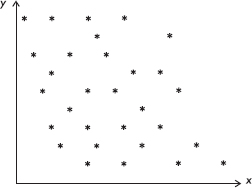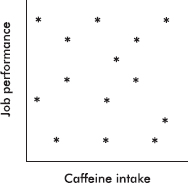# AP Statistics Multiple-Choice Practice Test 39

### Test Information10 questions23 minutes

1. Sampling error is

2. Suppose that the weights of trucks traveling on the interstate highway system are normally distributed. If 70% of the trucks weigh more than 12,000 pounds and 80% weigh more than 10,000 pounds, what are the mean and standard deviation for the weights of trucks traveling on the interstate system?

3. In the correlation coefficient r = 0.78, what percentage of variation in y is explained by the linear regression model?

4. Consider the following scatterplot:Which of the following is the best estimate of the correlation between x and y?

5. For one NBA playoff game the actual percentage of the television viewing public who watched the game was 24%. If you had taken a survey of 50 television viewers that night and constructed a confidence interval estimate of the percentage watching the game, which of the following would have been true?

I.The center of the interval would have been 24%.

II.The interval would have contained 24%.

III.A 99% confidence interval estimate would have contained 24%.

6. Which of the following is a true statement?

7. Consider the following scatterplot showing the relationship between caffeine intake and job performance.Which of the following is a reasonable conclusion?

8. An author of a new book claims that anyone following his suggested diet program will lose an average of 2.8 pounds per week. A researcher believes that the true figure will be lower and plans a test involving a random sample of 36 overweight people. She will reject the author's claim if the mean weight loss in the volunteer group is less than 2.5 pounds per week. Assume that the standard deviation among individuals is 1.2 pounds per week. If the true mean value is 2.4 pounds per week, what is the probability that the researcher will mistakenly fail to reject the author's false claim of 2.8 pounds?

9. Which of the following is the central limit theorem?

10. What is a sampling distribution?